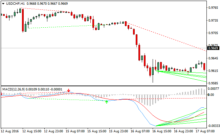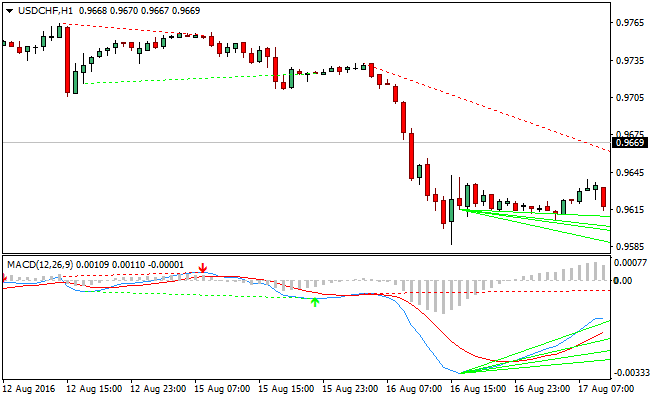# MACD Histogram Forex Indicator For MetaTrader 4The MACD Histogram forex indicator for MetaTrader 4 is a technical tool that is used by chartist to gauge the distance between the MACD and its signal line i.e. the 9-day EMA of MACD. The MACD_Histogram is equally an oscillator that swings above and below the zero signal line.

The MACD_Histogram indicator was developed by Thomas Aspray in 1986 to forecast signal crossovers in MACD. Although, the signal line crossover can come late due to the lagging nature of the MACD, which can hamper the reward-to-risk ration of a position.

Example ChartJust as we have with the MACD, the MACD_Histogram is also a nice tool that can be used in spotting divergence, convergence and crossovers. The MACD_Histogram does gauge the distance between the MACD and its signal line.

When the MACD is above its signal line the histogram is positive. As the MACD diverges away from its signal line, the positive values increase, but the values decreases when the MACD converges with its signal line.

When the MACD crosses below its signal line, the MACD_Histogram also crosses the zero line. If the MACD stays below its signal line, it indicates that the indicator is negative. When the MACD diverges from its signal line it denotes increasing negative values.

At the same time, when the MACD converges on its signal line, it signals decreasing negative values.

MT4 Indicator Characteristics

Currency pairs: Any